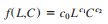### Create an Account

Home / Questions / The Cobb Douglas production function is a classic model from economics used to model outpu...

# The Cobb Douglas production function is a classic model from economics used to model output as a function of capital and labor It has the form where

The Cobb-Douglas production function is a classic model from economics used to model output as a function of capital and labor. It has the formwhere c0, c1, and c2 are constants. The variable L represents the units of input of labor, and the variable C represents the units of input of capital.

a. In this example, assume

c0 5 5, c1 5 0.25, and c2 5 0.75. Assume each unit of labor costs \$25 and each unit of capital costs \$75. With \$75,000 available in the budget, develop an optimization model to determine how the budgeted amount should be allocated between capital and labor in order to maximize output.

b. Find the optimal solution to the model you formulated in part a.

Apr 25 2020 View more View LessSubscribe To Get Solution